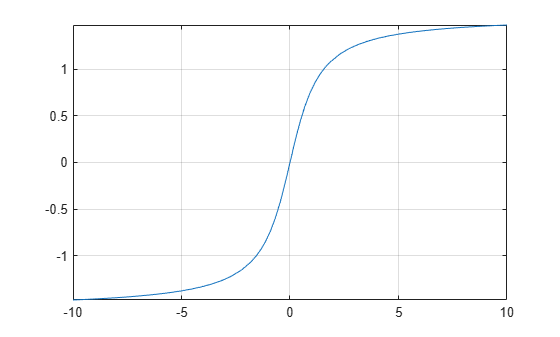atan

Symbolic inverse tangent function

Description

example

atan(X) returns the inverse tangent function (arctangent function) of X. All angles are in radians.

• For real values of X, atan(X) returns values in the interval [-pi/2,pi/2].

• For complex values of X, atan(X) returns complex values with the real parts in the interval [-pi/2,pi/2].

Examples

Inverse Tangent Function for Numeric and Symbolic Arguments

Depending on its arguments, atan returns floating-point or exact symbolic results.

Compute the inverse tangent function for these numbers. Because these numbers are not symbolic objects, atan returns floating-point results.

A = atan([-1, -1/3, -1/sqrt(3), 1/2, 1, sqrt(3)])
A =
-0.7854   -0.3218   -0.5236    0.4636    0.7854    1.0472

Compute the inverse tangent function for the numbers converted to symbolic objects. For many symbolic (exact) numbers, atan returns unresolved symbolic calls.

symA = atan(sym([-1, -1/3, -1/sqrt(3), 1/2, 1, sqrt(3)]))
symA =
[ -pi/4, -atan(1/3), -pi/6, atan(1/2), pi/4, pi/3]

Use vpa to approximate symbolic results with floating-point numbers:

vpa(symA)
ans =
[ -0.78539816339744830961566084581988,...
-0.32175055439664219340140461435866,...
-0.52359877559829887307710723054658,...
0.46364760900080611621425623146121,...
0.78539816339744830961566084581988,...
1.0471975511965977461542144610932]

Plot Inverse Tangent Function

Plot the inverse tangent function on the interval from -10 to 10.

syms x
fplot(atan(x),[-10 10])
grid onHandle Expressions Containing Inverse Tangent Function

Many functions, such as diff, int, taylor, and rewrite, can handle expressions containing atan.

Find the first and second derivatives of the inverse tangent function:

syms x
diff(atan(x), x)
diff(atan(x), x, x)
ans =
1/(x^2 + 1)

ans =
-(2*x)/(x^2 + 1)^2

Find the indefinite integral of the inverse tangent function:

int(atan(x), x)
ans =
x*atan(x) - log(x^2 + 1)/2

Find the Taylor series expansion of atan(x):

taylor(atan(x), x)
ans =
x^5/5 - x^3/3 + x

Rewrite the inverse tangent function in terms of the natural logarithm:

rewrite(atan(x), 'log')
ans =
(log(1 - x*1i)*1i)/2 - (log(1 + x*1i)*1i)/2

Input Arguments

collapse all

Input, specified as a symbolic number, variable, expression, or function, or as a vector or matrix of symbolic numbers, variables, expressions, or functions.